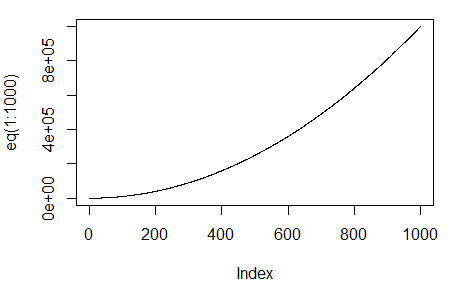# How to plot a function curve in R

1 view

What are the alternatives for drawing a simple curve for a function like

eq = function(x){x*x}

in R?

by (25.4k points)
edited

To plot a function curve in R, you can so the following:

eq = function(x){x*x}

plot(eq(1:1000), type='l')If you want to learn more about R programming watch this tutorial on Introduction to Data Science with R